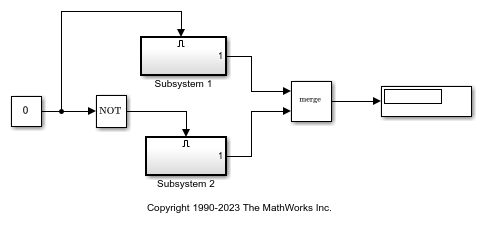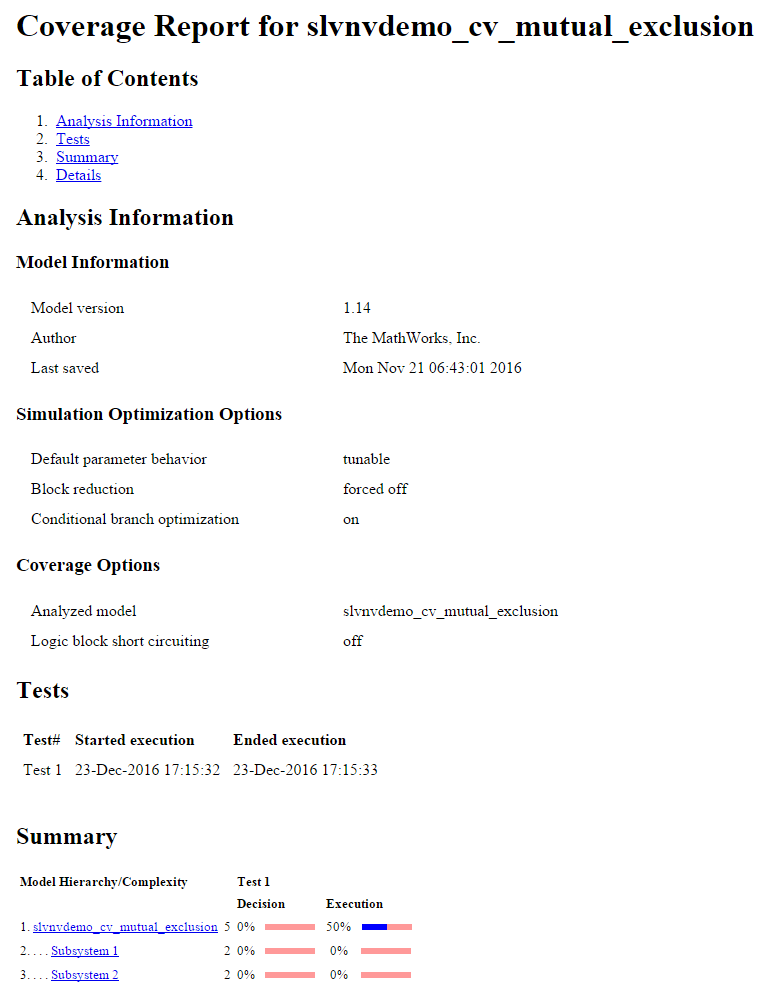# Perform Operations on Coverage Data

This example shows how to use the overloaded operators `+`, `*`, and `-` to combine coverage results into a union, intersection, or set difference of results.

### Open Model

Open the `slvnvdemo_cv_mutual_exclusion` model. The model contains a Constant block connected to the enable port of two subsystems. One of the subsystems has a `NOT` block placed before the enable port, which means only one of the subsystems can be enabled at a time.

```modelName = "slvnvdemo_cv_mutual_exclusion"; open_system(modelName) ```Create a `Simulink.SimulationInput` object and then use `setModelParameter` to set some coverage parameters.

```simIn = Simulink.SimulationInput(modelName); ```

Enable coverage analyze for the model by setting the `CovEnable` parameter to `on`.

```simIn = setModelParameter(simIn,"CovEnable","on"); ```

Set the structural coverage level to `Decision`.

```simIn = setModelParameter(simIn,"CovMetricStructuralLevel","Decision"); ```

Display the coverage data in MATLAB® instead of the file location by setting `CovSaveOutputData` to `off`. This also prevents Simulink® Coverage® from automatically saving the coverage data to a file after the simulation.

```simIn = setModelParameter(simIn,"CovSaveOutputData","off"); ```

Simulate the model by using `simIn` as the input to `sim`. The `SimulationOutput` object contains the coverage data as a property whose name is equal to the value of the `CovSaveName` configuration parameter. The default value is `covdata`.

```simOut1 = sim(simIn); data1 = simOut1.covdata ```
```data1 = ... cvdata version: (R2023b) id: 510 type: TEST_DATA test: cvtest object rootID: 512 checksum: [1x1 struct] modelinfo: [1x1 struct] startTime: 19-Aug-2023 15:34:32 stopTime: 19-Aug-2023 15:34:32 intervalStartTime: 0 intervalStopTime: 0 simulationStartTime: 0 simulationStopTime: 10 filter: simMode: Normal ```

Change which subsystem is enabled by using the `setBlockParameter` method to change the value of the Constant block from `0` to `1`, and then simulate the model again.

```simIn = setBlockParameter(simIn,modelName+"/Constant","value","1"); simOut2 = sim(simIn); data2 = simOut2.covdata ```
```data2 = ... cvdata version: (R2023b) id: 563 type: TEST_DATA test: cvtest object rootID: 512 checksum: [1x1 struct] modelinfo: [1x1 struct] startTime: 19-Aug-2023 15:34:32 stopTime: 19-Aug-2023 15:34:32 intervalStartTime: 0 intervalStopTime: 0 simulationStartTime: 0 simulationStopTime: 10 filter: simMode: Normal ```

Use the `decisioninfo` function to extract the decision coverage from each simulation and determine the percentage of decision outcomes satisfied.

```cov1 = decisioninfo(data1,modelName); percent1 = 100*(cov1(1)/cov1(2)) cov2 = decisioninfo(data2,modelName); percent2 = 100*(cov2(1)/cov2(2)) ```
```percent1 = 50 percent2 = 50 ```

Both simulations have 50% coverage. To check if the two simulations cover the same 50% of decision outcomes, look at the union and intersection of the two objects.

### Find the Union of Coverage

Use the `+` operator to derive a third `cvdata` object that represents the union of `data1` and `data2` `cvdata` objects. The union of two or more `cvdata` objects is also referred to as cumulative coverage or aggregated coverage.

When you create `cvdata` objects by combining other simulation results, the `type` property of the new object is `DERIVED_DATA`.

```dataUnion = data1 + data2 ```
```dataUnion = ... cvdata version: (R2023b) id: 0 type: DERIVED_DATA test: [] rootID: 512 checksum: [1x1 struct] modelinfo: [1x1 struct] startTime: 19-Aug-2023 15:34:32 stopTime: 19-Aug-2023 15:34:32 intervalStartTime: 0 intervalStopTime: 0 filter: simMode: Normal ```

Extract the decision coverage results and determine the percentage of decision outcomes satisfied by calling the `decisioninfo` function on `dataUnion`.

```covU = decisioninfo(dataUnion,modelName); percentU = 100*(covU(1)/covU(2)) ```
```percentU = 100 ```

The union of the two simulations reports that 100% decision outcomes are satisfied. This result indicates that there is no overlap in the coverage between the two simulations and that all decision outcomes are satisfied.

### Find the Intersection of Coverage

Confirm that the coverage does not overlap between the two simulations by intersecting `data1` and `data2` with the `*` operator. The intersection returns only the coverage outcomes that are satisfied in both `cvdata` objects.

```dataIntersection = data1 * data2 covI = decisioninfo(dataIntersection,modelName); percentI = 100*(covI(1)/covI(2)) ```
```dataIntersection = ... cvdata version: (R2023b) id: 0 type: DERIVED_DATA test: [] rootID: 512 checksum: [1x1 struct] modelinfo: [1x1 struct] startTime: 19-Aug-2023 15:34:32 stopTime: 19-Aug-2023 15:34:32 intervalStartTime: 0 intervalStopTime: 0 filter: simMode: Normal percentI = 0 ```

There is 0% decision coverage in the intersection because there is no overlap in coverage between the two simulations.

### Compute the Coverage Difference

Use the `-` operator to create a `cvdata` object that represents the set difference between the left and right operands. The result of the operation contains the coverage outcomes that are satisfied in the left operand but not satisfied in the right operand. Use this operation to determine how much additional coverage is attributed to a particular simulation.

In this example, the difference between the union of the first and second simulation coverage and the first simulation coverage indicates how much additional coverage the second simulation provided. Because none of the decision coverage outcomes overlapped, the new decision coverage from the second simulation is 50%.

```newCov2 = dataUnion - data1 covN = decisioninfo(newCov2,'slvnvdemo_cv_mutual_exclusion'); percentN = 100*(covN(1)/covN(2)) ```
```newCov2 = ... cvdata version: (R2023b) id: 0 type: DERIVED_DATA test: [] rootID: 512 checksum: [1x1 struct] modelinfo: [1x1 struct] startTime: 19-Aug-2023 15:34:32 stopTime: 19-Aug-2023 15:34:32 intervalStartTime: 0 intervalStopTime: 0 filter: simMode: Normal percentN = 50 ```

### Use Derived Coverage Data Objects

You can use derived `cvdata` objects in all reporting and analysis functions and as inputs to subsequent operations. For example, generate a coverage report from the derived `dataIntersection` object and create a new `cvdata` union.

```cvhtml('intersect_cov', dataIntersection); newUnion = dataUnion + dataIntersection ```
```newUnion = ... cvdata version: (R2023b) id: 0 type: DERIVED_DATA test: [] rootID: 512 checksum: [1x1 struct] modelinfo: [1x1 struct] startTime: 19-Aug-2023 15:34:32 stopTime: 19-Aug-2023 15:34:32 intervalStartTime: 0 intervalStopTime: 0 filter: simMode: Normal ```